# CAD > Insert > Circle

A circle is a model entity which is graphically defined by three associated points. These three associated points define the origin of the circle, the length of its first axis and the length of its second axis respectively. The first axis point is also the start/endpoint of the circle (i.e. the start/end of its circumference) and the second axis point is always located at right angles to it with respect to the origin, in a direction dependent upon the method of insertion and the current CPL.

A circle can be inserted freely or relative to existing entities (e.g. tangentially). The lengths of the axes of a circle are equal. An ellipse can be created by specifying different lengths for the axes. A circle can subsequently be trimmed and divided, and points can be generated around the circumference.   Not all these options are available from the Pulldown Menu. For the rest of the options, turn on the right hand menu and go to CAD > Circle.

1. The visual display of circles and arcs can be suppressed by selecting the No option for the Circles modifier of the Defaults Vis-Model command.
2. The three associated points of a circle can be selected with the snap/help button for exact co-ordinate location but they cannot be deleted.

Circle Insert

The Circle Insert command enables the Circle Insert sub-menu which allows the insertion of circles by defining point locations, radii, diameters or curvature. Circles tangential to specific existing entities and perpendicular to an existing line can also be inserted. Ellipses can also be inserted by defining point locations or axis lengths.

3-Points
Allows the insertion of a circle whose circumference passes through three selected point locations. The first point location selected is the start/end of the circle’s circumference. Together with this first point location, the next two point locations selected define the size and orientation of the circle.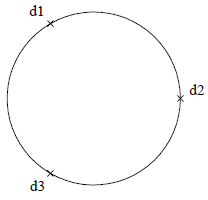If the three locations selected are co-linear, a circle will not be inserted.

Allows the insertion of a circle whose radius is defined by selecting two point locations.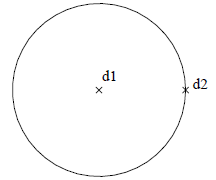The first point selected is the origin of the circle; the second point is the start/endpoint of the circle, i.e. a point on the circumference of the circle.

Allows the insertion of a circle with a specified radius.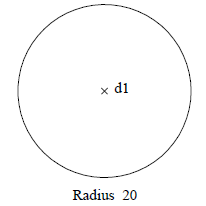The radius is specified, followed by the selection of the origin of the circle. The orientation of the circle is determined by the current CPL.

Diam
Allows the insertion of a circle whose diameter is defined by selecting two point locations.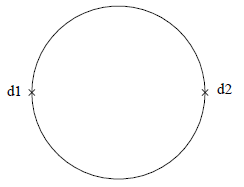The first point location selected is the start/endpoint of the circle and the second point location selected defines the diameter and orientation of the circle (i.e. distance and direction between the first and second selected point locations).

Diam=
Allows the insertion of a circle with a specified diameter.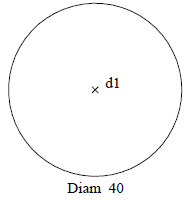The diameter is specified, followed by the selection of the origin of the circle. The orientation of the circle is determined by the current CPL.

Tang-Lin-Pnt
Allows the insertion of a circle with a specified radius that is tangential to an existing line and has its circumference passing through a selected point location. The radius is specified followed by the selection of the reference line. The reference point location is then selected followed by the selection of the approximate origin of the circle.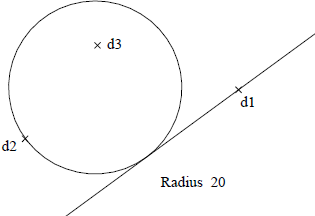This results in a circle with the specified radius being inserted tangential to the reference line where its circumference passes through the reference point location. The start/endpoint of the circle is its tangent point on the reference line and the orientation of the circle is determined by this tangent point and the reference point location.

This command regards the reference line as having infinite endpoint extensions when determining its point of tangency with the resultant circle.

Tang-Lin-Lin
Allows the insertion of a circle with a specified radius that is tangential to two intersecting lines.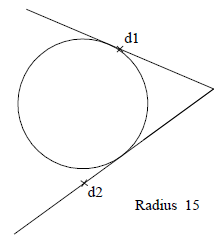The radius is specified followed by the selection of the two reference lines near the ends from which the respective tangent points are to be calculated. This results in a circle with a specified radius being inserted tangential to the reference lines by calculating where it has tangent points on both lines simultaneously. Thus, the origin of the circle is placed on the bisector of the angle created by the two reference lines.

1. Reference lines are regarded as having infinite endpoint extensions to determine their points of intersection and their points of tangency with the resultant circle.
2. Lines are 3D model entities. Although they may appear co-planar in the current view, they may not actually be co-planar in 3D space. Although they may appear to intersect in the current view, they may not actually intersect in 3D space.

Tangent 3 lines
Allows the insertion of a circle that is tangential to three co-planar lines. The three reference lines are selected near the ends from which the respective tangent points are to be calculated, followed by the selection of a point location which defines the approximate origin point of the desired tangential circle in relation to the reference lines.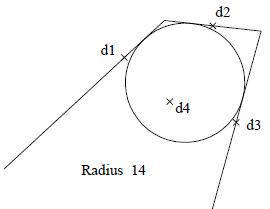This results in a circle being inserted tangential to the reference lines by calculating where it has tangent points on all lines simultaneously. It circumference will pass through all tangent points. The start/endpoints of the circle is the tangent point on the first reference line selected. The orientation of the circle is determined by the tangent points of the respectively selected reference lines.

1. All three reference lines must not be parallel.
2. None of the three reference lines can be co-linear.
3. This command regards the reference lines as having infinite endpoint extensions when determining their points of intersection and their points of tangency with the resultant circle.
4. Lines are 3D model entities. Although they may appear co-planar in the current view, they may not actually be co-planar in 3D space.

Tangent 3 circles
Allows the insertion of a circle that is tangential to three co-planar circles. The three reference circles are selected approximately at the tangential point of the desired circle.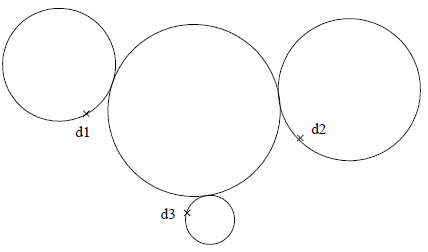This results in a circle being inserted tangential to the three reference circles, by calculating where it has tangent points on all three circles simultaneously. The circumference of the resultant circle will pass through all three tangent points. The Three reference circles must be co-planar.